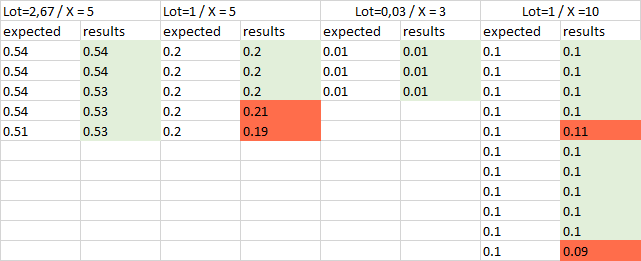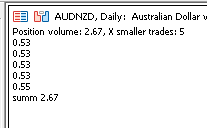# split up lot

Hello
I'm facing a problem, and I need some help.

I'm trying to code this feature:  "Split up trade into X smaller trades "
i.e the user  can set 1 standart lot and set 5 to this parameter. (In this case, the ea should open 5 trades with 0.20 lot size)

This the code i wrote

```void getSplittedVolume(double volume, int splitX, double &newVolumes[])
{
double lotStep = m_symbol.LotsStep();
int divisor=splitX;
for(int step=divisor; step>0; step--)
{
int size_position=ArraySize(newVolumes);
double size;
if (volume <DBL_EPSILON)  continue;
if (step == 1)
size=volume;
else
size=MathCeil(volume/step/lotStep)*lotStep;

volume -= size;
if(size>DBL_EPSILON)
{
ArrayResize(newVolumes,size_position+1);
newVolumes[size_position]=size;
}
}
}```

This code give sometimes inconsistent results. I think it has to do with how floating point numbers are handled.Can anyone guide me on how to fix/improve  my code to get the expected smaller lots

William Roeder #:

Sorry, I'm a beginner. I do not understand how the link you sent can help me to have the correct size.

bodojan468 #: I do not understand how the link you sent can help me to have the correct size.

MT4: Learn to code it.
MT5: Begin learning to code it.

If you don't learn MQL4/5, there is no common language for us to communicate. If we tell you what you need, you can't code it. If we give you the code, you don't know how to integrate it into your code.

or pay (Freelance) someone to code it. Top of every page is the link Freelance.
Hiring to write script - General - MQL5 programming forum (2019)

We're not going to code it for you (although it could happen if you are lucky or the problem is interesting.) We are willing to help you when you post your attempt (using CODE button) and state the nature of your problem.
No free help (2017)

William Roeder #:

MT4: Learn to code it.
MT5: Begin learning to code it.

If you don't learn MQL4/5, there is no common language for us to communicate. If we tell you what you need, you can't code it. If we give you the code, you don't know how to integrate it into your code.

or pay (Freelance) someone to code it. Top of every page is the link Freelance.
Hiring to write script - General - MQL5 programming forum (2019)

We're not going to code it for you (although it could happen if you are lucky or the problem is interesting.) We are willing to help you when you post your attempt (using CODE button) and state the nature of your problem.
No free help (2017)

Sorry, but that doesn't help me.
The code in the link you sent to me is similar to mine. I'm not asking you to code it for me I'm asking for tips, advice...

I faced to a similar problem long time ago.

You can use this tips : use integer instead of double.
If your lot size is 2.67  or  1 or... transform it in 267 or 100 or ...  then adapt your code

Cheers

I offer my version. What it does: This code corrects the last number.

```//+------------------------------------------------------------------+
//|                      https://www.mql5.com/en/users/barabashkakvn |
//+------------------------------------------------------------------+
#property version   "1.00"
//---
//---
CSymbolInfo    m_symbol;                     // object of CSymbolInfo class
//--- input parameters
input double   InpVolume   = 2.67;  // Position volume (ONLY TWO DIGITS after the comma!)
input uchar    InpXSmaller = 5;     // X smaller trades
//+------------------------------------------------------------------+
//| Expert initialization function                                   |
//+------------------------------------------------------------------+
int OnInit()
{
ResetLastError();
if(!m_symbol.Name(Symbol())) // sets symbol name
{
Print(__FILE__," ",__FUNCTION__,", ERROR: CSymbolInfo.Name");
return(INIT_FAILED);
}
//---
return(INIT_SUCCEEDED);
}
//+------------------------------------------------------------------+
//| Expert deinitialization function                                 |
//+------------------------------------------------------------------+
void OnDeinit(const int reason)
{
//---
}
//+------------------------------------------------------------------+
//| Expert tick function                                             |
//+------------------------------------------------------------------+
void OnTick()
{
double smaller_array[];
ArrayResize(smaller_array,InpXSmaller);
//---
for(int i=0; i<InpXSmaller; i++)
{
smaller_array[i]=LotCheck(InpVolume/((double)InpXSmaller),m_symbol);
}
//---
string text="Position volume: "+DoubleToString(InpVolume,2)+", X smaller trades: "+IntegerToString(InpXSmaller);;
double summ=0.0;
for(int i=0; i<InpXSmaller-1; i++)
{
text=text+"\n"+DoubleToString(smaller_array[i],2);
summ+=smaller_array[i];
}
smaller_array[InpXSmaller-1]=LotCheck(InpVolume-summ,m_symbol);
summ+=smaller_array[InpXSmaller-1];
text=text+"\n"+DoubleToString(smaller_array[InpXSmaller-1],2);
Comment(text,"\n","summ ",DoubleToString(summ,2));
}
//+------------------------------------------------------------------+
//| Lot Check                                                        |
//+------------------------------------------------------------------+
double LotCheck(double lots,CSymbolInfo &symbol)
{
//--- calculate maximum volume
double volume=NormalizeDouble(lots,2);
double stepvol=symbol.LotsStep();
if(stepvol>0.0)
volume=stepvol*MathFloor(volume/stepvol);
//---
double minvol=symbol.LotsMin();
if(volume<minvol)
volume=0.0;
//---
double maxvol=symbol.LotsMax();
if(volume>maxvol)
volume=maxvol;
//---
return(volume);
}
//+------------------------------------------------------------------+
```

Result:Files:

Mike Pascal Plavonil #:

I faced to a similar problem long time ago.

You can use this tips : use integer instead of double.
If your lot size is 2.67  or  1 or... transform it in 267 or 100 or ...  then adapt your code

Cheers

Hello

You rock's man. That was very helpful